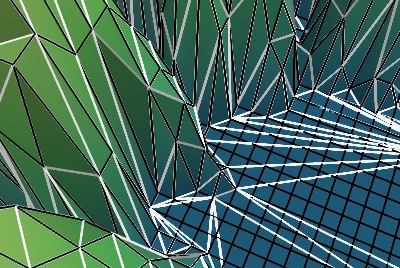# Julia set decimation example¶

The Julia set, but with a decimated mesh: unnecessary triangles due to the initial grid and not matching the geometry of the Julia set are removed.

We first build the mesh, applying a warp_scalar filter to a array2d_source, to warp the Julia set along the z direction.

Then when have to convert the rectangles in the mesh to triangles, in order to apply the decimate_pro filter. This filter does the decimation, and we can represent the result using surface modules.

The triangle-generation filter generates warnings: some polygons are degenerate, as the grid has subdivided flat parts of the Julia set.

We have shown in white the decimated mesh, and in black the non-decimated one. The view is zoom to the center of the Julia set. If you turn of the wireframes and zoom out, you can appreciate the quality of the decimation.

In the specific case of decimating a surface warped from 2D data, it is more efficient to use the greedy-terrain-decimator, see the Canyon decimation example.Python source code: `julia_set_decimation.py`

```
# Author: Gael Varoquaux <gael.varoquaux@normalesup.org>
# Copyright (c) 2008, Enthought, Inc.

from mayavi import mlab
import numpy as np

# Calculate the Julia set on a grid
x, y = np.ogrid[-1.5:0.5:500j, -1:1:500j]
z = x + 1j * y

julia = np.zeros(z.shape)

for i in range(50):
z = z ** 2 - 0.70176 - 0.3842j
julia += 1 / float(2 + i) * (z * np.conj(z) > 4)

mlab.figure(size=(400, 300))

# Create the mesh
mesh = mlab.pipeline.warp_scalar(mlab.pipeline.array2d_source(julia),
warp_scale=100)

# The decimate_pro filter works only on triangles. We need to apply the
# triangle_filter before applying decimate_pro.
dec = mlab.pipeline.decimate_pro(mlab.pipeline.triangle_filter(mesh))
# Set a very low feature_angle, so that the decimate_pro detects
dec.filter.feature_angle = 1
dec.filter.target_reduction = 0.5

# We display the lines of decimated mesh in white
mlab.pipeline.surface(dec, representation='wireframe', line_width=3,
color=(1, 1, 1))
# The decimated mesh itself.
mlab.pipeline.surface(dec, colormap='gist_earth', vmin=-0.1, vmax=0.4)

# The lines of the non-decimated mesh, in black, for comparisation.
mlab.pipeline.surface(mesh, representation='wireframe',  color=(0, 0, 0))

mlab.view(-66, 25, 9.7, [-5.8, -54.5,  18.4])

mlab.show()
```